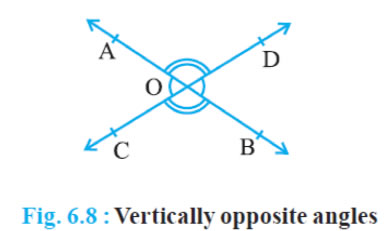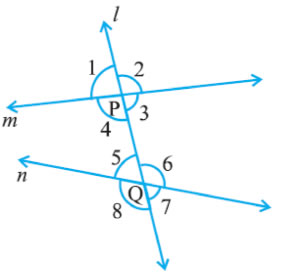# theorem of chap.6

theorem of chap.6

Gaurav Seth 2 weeks ago

Theorem 6. 1 : If two lines intersect each other, then the vertically opposite angles are equal
Given :- two lines intersect each other
To prove :-   ∠AOC =   ∠BOD  and ∠AOD =  ∠BOC

Proof  :- AB and CD be two lines intersecting at O as shown in Fig  6 8  They lead to two pairs of
vertically opposite angles, namely, (i)   ∠AOC and   ∠ BOD
(ii)   ∠ AOD and  ∠ BOCWe need to prove that   ∠AOC =   ∠BOD
and   ∠AOD =  ∠BOC
Now, ray OA stands on line CD
From (Linear pair axiom)
∠AOC +   ∠AOD = 180°     … (1)
∠AOD +   ∠BOD = 180°     … (2)
From (1) and (2), we can write
∠AOC +   ∠AOD =   ∠AOD +   ∠BOD
This implies that   ∠AOC =   ∠BOD
Similarly, it can be proved that  ∠AOD =  ∠BOC

Parallel Lines and a Transversal∠1, ∠ 2, ∠7 and  ∠ 8 are called exterior angles,
∠ 3,  ∠ 4,  ∠ 5 and   ∠6 are called interior angles
(a) Corresponding angles :
(i)   ∠1  =  ∠ 5 (ii)  ∠ 2  =  ∠ 6 (iii)  ∠ 4 =  ∠ 8 (iv)  ∠ 3 = ∠  7
(b) Alternate interior angles :
(i)  ∠ 4 =  ∠ 6 (ii)  ∠ 3 =  ∠ 5
(c) Alternate exterior angles:
(i)   ∠1 =  ∠ 7 (ii)  ∠ 2 =   ∠8
(d) Interior angles on the same side of the transversal:
(i)   ∠4 +   ∠5  =180°(ii)   ∠3  +  ∠ 6 =180°

Related Questions:## myCBSEguide

Trusted by 1 Crore+ Students

#### CBSE Test Generator

Create papers in minutes

Print with your name & Logo

3 Lakhs+ Questions

Solutions Included

Based on CBSE Blueprint

Best fit for Schools & Tutors

#### Work from Home

• Work from home with us
• Create questions or review them from home

No software required, no contract to sign. Simply apply as teacher, take eligibility test and start working with us. Required desktop or laptop with internet connection